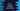# 2 different C++ program to check if a number is spy number or not## C++ program to check if a number is spy number or not:

In this C++ program, we will learn how to check if a number is a spy number or not. A number is called a spy number if the sum of the digits of the number is equal to the product of the digits. The program will take a number as an input from the user and check if the number is a spy number or not.

For example, 132 is a spy number. Because the sum of the digits of this number is 1 + 3 + 2 = 6 and the product of the digits of this number is 1 * 3 * 2 = 6. Both are equal.

### Algorithm:

We will use the below algorithm:

• Take the number as input from the user and save it to a variable.
• Initialize one variable as 0 to hold the sum of digits of the number. Initialize one more variable as 1 to hold the product of digits of the number.
• Run one loop till the number is greater than 0.
• On each iteration, get the last digit of the number by using modulo. Add this digit to the sum variable and multiply it to the product variable.
• Remove the last digit of the number by dividing it by 10.
• Once the loop ends, compare the sum variable with the product variable. If both are equal, this is a spy number. Else, it is not.

### Example 1: C++ program to check if a number is spy number or not:

The below program checks if a number is spy number or not:

``````#include <iostream>
using namespace std;

int main()
{
int num, sum = 0, product = 1, lastDigit;

cout << "Enter a number: " << endl;
cin >> num;

while (num > 0)
{
lastDigit = num % 10;
sum += lastDigit;
product *= lastDigit;

num /= 10;
}

if (sum == product)
{
cout << "It is a spy number !!" << endl;
}
else
{
cout << "It is not a spy number !!" << endl;
}
}``````

Here,

• Four integer variables are created in the beginning of the program. The variable num is used to store the number, sum is used to store the sum of digits of the number, product is used to store the product of the digits of the number, and lastDigit is used to store the last digit of the number.
• It asks the user to enter the number. This is assigned to the num varable.
• The while loop finds the sum and products of the digits of the number.
• On each iteration, it finds the last digit of the number and adds it to the sum variable. Also, it multiplies it to the product variable.
• At the end of each iteration, it removes the last digit of the number.
• Once the while loop ends, it compares the values of sum and product and prints one message.

If you run this program, it will print output as like below:

``````Enter a number:
132
It is a spy number !!

Enter a number:
133
It is not a spy number !!``````

### Example 2: C++ program to check if a number is spy number or not by using a separate function:

Let’s use a separate function to find out if a number is spy number or not. This function will take one number as the parameter and return one boolean value.

``````#include <iostream>
using namespace std;

bool isSpy(int num)
{
int sum = 0, product = 1, lastDigit;
while (num > 0)
{
lastDigit = num % 10;
sum += lastDigit;
product *= lastDigit;

num /= 10;
}

return sum == product;
}

int main()
{
int num;

cout << "Enter a number: " << endl;
cin >> num;

if (isSpy(num))
{
cout << "It is a spy number !!" << endl;
}
else
{
cout << "It is not a spy number !!" << endl;
}
}``````
• isSpy is a separate function to check if a number is spy or not. It takes one number as the parameter and returns one boolean value: *true if the number is spy and false otherwise.
• isSpy is using the same process similar to the previous example to check if the provided number is spy or not.
• It returns one boolean value and based on it, the if-else block in the main method prints a message.

It will print similar output.

``````Enter a number:
133
It is not a spy number !!

Enter a number:
1412
It is a spy number !!``````